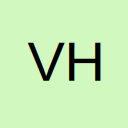# Staubli Appro and Compose

• Hi everyone,

I don't understand what the différence is between offset whit Appro and Compose. Can you help me please ? Thank you.

- VHS

• Hi,

They are very similar but totally differentAppro, will generate a position with a given offset according to the tool coordinate system.

point appro(point pPosition, trsf trTransformation)

When you put the trsf.z = -100 it will move to the position with an offset of -100 according to the tool coordenate system, see image below.• Compose, will generate a position with a given offset, relative to the given frame coordinate system.

point compose(point pPosition, frame fReference,trsf trTransformation)

When you put the trsf.z = -100 it will move to the position with an offset of -100 according to the tool given frame coordinate system, see image below.• Hi,

I am a beginner in robotics.

ElEsgalho According to your image, if I understood correctly, for XYZ it is therefore the same as adding 160 in X and 80 in Y to pFirst point relative to the frame fPallet?

p=compose(pFirst,fpallet,{160,80,0,0,0,0})

Can we assume that on the frame fPallet this calculation is the same as compose():

p.X = pfirst + 160

p.Y = pfirst + 80

p.Z = pfirst + 0

What about orientation Rx Ry and Rz ?

Please correct me if this is completely wrong• Hi,

I am a beginner in robotics.

Welcome aboardit is therefore the same as adding 160 in X and 80 in Y to pFirst point relative to the frame fPallet?

Yes, it's exactly as you said relative to the frame, it's important to know that is according to the frame coordinate system. Same for the rotation Rx,Ry,Rz.

p=compose(pFirst,fpallet,{160,80,0,0,0,0})

Can we assume that on the frame fPallet this calculation is the same as compose():

p.X = pfirst + 160

p.Y = pfirst + 80

p.Z = pfirst + 0

Well, it depends if p is in the same frame, if so, adding would be the same.

If for example, that point p was in the world frame, adding the value of pfirst + offset would go in the X direction of the world frame, the resulting point would be different that the compose. If on the same frame, yes is the same composing or adding.

Also, when manipulating points with VAL3 the way to get access to the X value of a point is like this:

Code
``p.trsf.x=pfirst.trsf.x+160``

The point variable has 2 fields, the configuration "config" and the transformation "trsf", so if you want to change or get access to the positional values, please first get access to the transformation.

• Hello,

+1 to EiEsgalho.

In reality, the APPRO function don't work on the TCP but on the POINT. It's the reason why you don't need to indicate, in the calcul, the tool name. When your robot is in the position, the TCP and the POINT are in the same position,so it's easier to say that it work arround the TCP.

Very often the Z value of the offset is negative.

With COMPOSE, the result take the frame of the reference point (in the #4 image, p's frame is pFirst's frame) , but the offset can be in other frame ( #4 : fPallet). Often, Reference point's frame is the offset frame.

If the frame Z axis is to the top, the Z value is often positive !

To use COMPOSE is clearer to modifie the values...and fasterQuote

What about orientation Rx Ry and Rz ?

You can rotate your point arrount the reference point with an offset like {0,0,0,10,0,0} (turn 10 degrees arround Rx). It's possible with the both functions. Be carreful that Stäubli calculate all the points, tools, and Frame, in the order :

1- Tx, TY,TZ

2- Rx

3- RY

4- RZ

Some other materlal (like Fanuc or Kuka) don't calculate in the same order !

Have a good day...

Edited 2 times, last by Galet ().